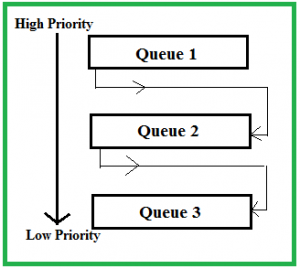Open in App
Not now

# Multilevel Feedback Queue Scheduling (MLFQ) CPU Scheduling

• Difficulty Level : Easy
• Last Updated : 13 Jul, 2022

Multilevel Feedback Queue Scheduling (MLFQ) CPU Scheduling is like Multilevel Queue(MLQ) Scheduling but in this processes can move between the queues. And thus, much more efficient than multilevel queue scheduling.

Characteristics of Multilevel Feedback Queue Scheduling:

• In a multilevel queue-scheduling algorithm, processes are permanently assigned to a queue on entry to the system and processes are not allowed to move between queues.
• As the processes are permanently assigned to the queue, this setup has the advantage of low scheduling overhead,
• But on the other hand disadvantage of being inflexible.

Advantages of Multilevel Feedback Queue Scheduling:

• It is more flexible.
• It allows different processes to move between different queues.
• It prevents starvation by moving a process that waits too long for the lower priority queue to the higher priority queue.

Disadvantages of Multilevel Feedback Queue Scheduling:

• For the selection of the best scheduler, it requires some other means to select the values.
• It produces more CPU overheads.
• It is the most complex algorithm.

Multilevel feedback queue scheduling, however, allows a process to move between queues. Multilevel Feedback Queue Scheduling (MLFQ) keeps analyzing the behavior (time of execution) of processes and according to which it changes its priority.

Now, look at the diagram and explanation below to understand it properly.Now let us suppose that queues 1 and 2 follow round robin with time quantum 4 and 8 respectively and queue 3 follow FCFS.

Implementation of MFQS is given below –

• When a process starts executing the operating system can insert it into any of the above three queues depending upon its priority. For example, if it is some background process, then the operating system would not like it to be given to higher priority queues such as queue 1 and 2. It will directly assign it to a lower priority queue i.e. queue 3. Let’s say our current process for consideration is of significant priority so it will be given queue 1.
• In queue 1 process executes for 4 units and if it completes in these 4 units or it gives CPU for I/O operation in these 4 units then the priority of this process does not change and if it again comes in the ready queue then it again starts its execution in Queue 1.
• If a process in queue 1 does not complete in 4 units then its priority gets reduced and it shifted to queue 2.
• Above points 2 and 3 are also true for queue 2 processes but the time quantum is 8 units. In a general case if a process does not complete in a time quantum then it is shifted to the lower priority queue.
• In the last queue, processes are scheduled in an FCFS manner.
• A process in a lower priority queue can only execute only when higher priority queues are empty.
• A process running in the lower priority queue is interrupted by a process arriving in the higher priority queue.

Well, the above implementation may differ for example the last queue can also follow Round-robin Scheduling.

Problems in the above implementation: A process in the lower priority queue can suffer from starvation due to some short processes taking all the CPU time.

Solution: A simple solution can be to boost the priority of all the processes after regular intervals and place them all in the highest priority queue.

### What is the need for such complex Scheduling?

• Firstly, it is more flexible than multilevel queue scheduling.
• To optimize turnaround time algorithms like SJF are needed which require the running time of processes to schedule them. But the running time of the process is not known in advance. MFQS runs a process for a time quantum and then it can change its priority(if it is a long process). Thus it learns from past behavior of the process and then predicts its future behavior. This way it tries to run a shorter process first thus optimizing turnaround time.
• MFQS also reduces the response time.

Example: Consider a system that has a CPU-bound process, which requires a burst time of 40 seconds. The multilevel Feed Back Queue scheduling algorithm is used and the queue time quantum ‘2’ seconds and in each level it is incremented by ‘5’ seconds. Then how many times the process will be interrupted and in which queue the process will terminate the execution?

Solution:

• Process P needs 40 Seconds for total execution.
• At Queue 1 it is executed for 2 seconds and then interrupted and shifted to queue 2.
• At Queue 2 it is executed for 7 seconds and then interrupted and shifted to queue 3.
• At Queue 3 it is executed for 12 seconds and then interrupted and shifted to queue 4.
• At Queue 4 it is executed for 17 seconds and then interrupted and shifted to queue 5.
• At Queue 5 it executes for 2 seconds and then it completes.
• Hence the process is interrupted 4 times and completed on queue 5.

This article is contributed by Ashish Sharma. If you like GeeksforGeeks and would like to contribute, you can also write an article using write.geeksforgeeks.org or mail your article to review-team@geeksforgeeks.org. See your article appearing on the GeeksforGeeks main page and help other Geeks.

My Personal Notes arrow_drop_up